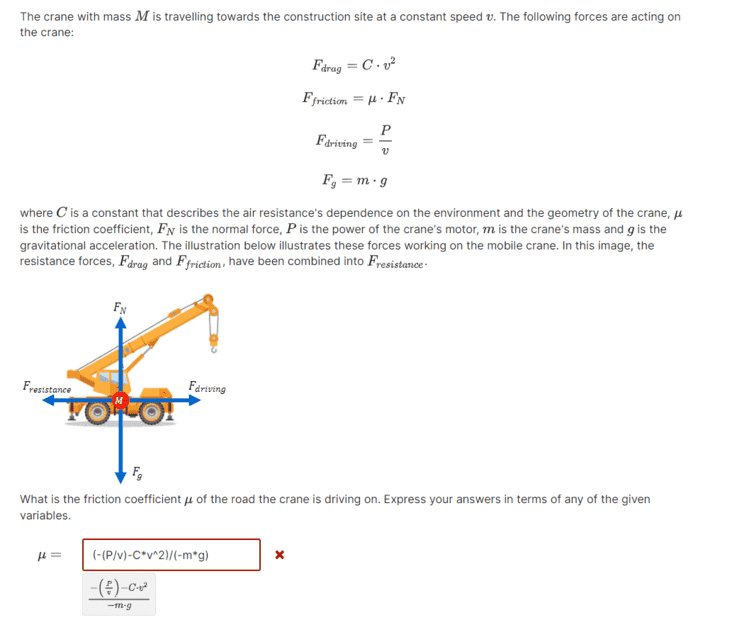# Forces acting on a moving crane

Thickmax
Homework Statement:
Engineering course top up physics module
Relevant Equations:
See belowI've checked my answer many times and do not understand what I am missing

Paulpaulpa
You probably made an error with signs. Remember that ##F_{resistance} \propto - \vec{e_x}## while ##F_{driving} \propto \vec{e_x}##.

•Delta2
Thickmax
1/(M*g*v)*(P-C*v^3)

No idea how this is the case

•Delta2
Paulpaulpa
1/(M*g*v)*(P-C*v^3)

No idea how this is the case

Since ##\vec{F}_{total}.\vec{e}_y = 0##, we have $$\vec{F}_{N} = mg \vec{e}_y$$ $$\vec{F}_{Friction} = - \mu mg \vec{e_x}$$ and $$\vec{F}_{drag} = - C v^2 \vec{e}_x$$ As you did for your answer you then take ##\vec{F}_{total}.\vec{e}_x = 0## adn resolve the equation for ##\mu##.

•Thickmax
Homework Helper
Gold Member
The crane is moving with constant horizontal velocity. Hence the vector sum of the horizontal forces is zero, that is
$$\vec{F}_{resistance}+\vec{F}_{driving}=\vec{0}$$

In order to transform the above vector equation to an algebraic equation , you have to be careful with signs and conventions. If we make the convention that the positive direction is towards right , then $$\vec{F}_{driving}=\frac{P}{v}\hat x$$ that is it is positive force, but $$\vec{F}_{resistance}=-Cv^2\hat x-\mu mg\hat x$$, that is it is negative (towards the left).
Thus the initial vector equation will transform to the algebraic equation
$$-Cv^2-\mu mg+\frac{P}{v}=0$$ which you can solve for ##\mu## and you will get what you saying at post #3.

•Lnewqban and Thickmax
Thickmax
The crane is moving with constant horizontal velocity. Hence the vector sum of the horizontal forces is zero, that is
$$\vec{F}_{resistance}+\vec{F}_{driving}=\vec{0}$$

In order to transform the above vector equation to an algebraic equation , you have to be careful with signs and conventions. If we make the convention that the positive direction is towards right , then $$\vec{F}_{driving}=\frac{P}{v}\hat x$$ that is it is positive force, but $$\vec{F}_{resistance}=-Cv^2\hat x-\mu mg\hat x$$, that is it is negative (towards the left).
Thus the initial vector equation will transform to the algebraic equation
$$-Cv^2-\mu mg+\frac{P}{v}=0$$ which you can solve for ##\mu## and you will get what you saying at post #3.
Thanks Very much!

But I'm struggling to simplify the last equation still.

-(Cv^2)-(UMG)+(P/v)=0 = -(Cv^2)+(P/V)=UMG

U=(-(Cv^2)+(P/v))/(MG)

Am I missing something?

Homework Helper
Gold Member
Am I missing something?

No you got it right, you just need one last algebraic step: multiply both the numerator and the denominator of the fraction by v and then you 'll get what you say at post #3.

•Thickmax
Thickmax
No you got it right, you just need one last algebraic step: multiply both the numerator and the denominator of the fraction by v and then you 'll get what you say at post #3.
How do you know when to do that?Is this method called something that i can read up on?

Many thanks again

•Delta2
Homework Helper
Gold Member
How do you know when to do that?Is this method called something that i can read up on?
Ehm its fundamental algebraic rule: IF you multiply both the numerator and the denominator of a fraction with the same thing, the fraction remains the same. That is $$\frac{a}{b}=\frac{ac}{bc}$$

•Thickmax
Thickmax
Ehm its fundamental algebraic rule: IF you multiply both the numerator and the denominator of a fraction with the same thing, the fraction remains the same. That is $$\frac{a}{b}=\frac{ac}{bc}$$
Oh I see now🤦‍♂️But how do you know when to do that? Is it just to tidy up the equation and have ‘one line’ as the numerator?

Homework Helper
Gold Member
Oh I see now🤦‍♂️But how do you know when to do that? Is it just to tidy up the equation and have ‘one line’ as the numerator?
Yes you can view it like this.

•Thickmax
Homework Helper
Gold Member
2022 Award
Oh I see now🤦‍♂️But how do you know when to do that? Is it just to tidy up the equation and have ‘one line’ as the numerator?
Yes, it is usual to avoid nested fractions in the simplified form. But more generally, you cannot assume the target form is what you would consider the simplest such. You have to be prepared to check whether there is some way of manipulating the one into the other.

•Thickmax and Delta2
Thickmax
Got it! thanks

But then why does the answer have (1/M*G*V)*(P-C*V^3)

Why can't it just be U=(-(CV^3)+(P))/(MGV) = (P-(CV^3))/(MGV)

Think this is a stupid question as I know the answer will be the same, but I don't understand how to know/why you simplify this far.

It doesn't help that I don't know all the rules either ;)

Homework Helper
Gold Member
$$\frac{1}{A}B=\frac{B}{A}$$

•Thickmax